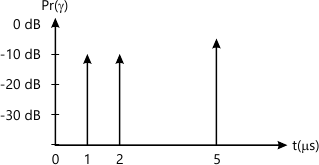MORE IN Antenna and Wave Propagation
SPPU Electronics and Telecom Engineering (Semester 6)
Antenna and Wave Propagation
June 2015
Total marks: --
Total time: --
INSTRUCTIONS
(1) Assume appropriate data and state your reasons
(2) Marks are given to the right of every question
(3) Draw neat diagrams wherever necessary

Answer any one question from Q1 and Q2
1 (a) What is polarization of wave? Explain the polarization of three types of wave with the help of relevant diagram?
8 M
1 (b) Write a short note on
i) Ionospheric abnormalities
ii) Multihop propagation
6 M
1 (c) A lossless resonant λ/2 dipole antenna with input impedance of 73Ω is to be connected to a transmission line whose characteristic impedance is 50Ω. Assuming that the pattern the antenna is given approximately by U = Bo sin3?. Find the overall maximum gain of this antenna.
6 M

2 (a) What is Poynting vector? What is its significance? Derive an expression for Poynting vector?
6 M
2 (b) Explain antenna radiation mechanism in detail.
6 M
2 (c) Calculate the mean excess delay, RMS delay spread, and the maximum excess delay (10dB) for the multipath profile given in the figure below. Estimate the 50% coherence bandwidth of the channel.8 M

Answer any one question from Q3 and Q4
3 (a) Derive the expression for radiation resistance of Infinitesimal Dipole.
9 M
3 (b) Derive the expression for radiation resistance of small dipole antenna.
9 M

4 (a) Calculate the radiation resistance of a double turn and an eight turn small circular loop when radius of loop is λ/10 and the medium is free spACe. Calculate its efficiency if loss resistance is 25Ω.
8 M
4 (b) Derive mathematical expression for power density and radiation intensity of half wave dipole antenna and draw radiation pattern of half wave dipole antenna in E and H plane.
10 M

Answer any one question from Q5 and Q6
5 (a) Write a short notes on
i) Pattern Multiplication.
ii) Binomial Array.
8 M
5 (b) Design a broad side Dolph-Tschebhysheff array of five elements with half wavelength spACing between elements and with major to minor lobe ratio to be 19dB. Find the excitation coefficients & array fACtor.
8 M

6 (a) Explain planar array. State its advantages and applications.
6 M
6 (b) An Endfire array with element spACed at λ/2 and with axes of elements at right angles to the line of array is required to have directivity of 36. Determine -the array length and the width of major lobe.
5 M
6 (c) Give the comparison of broadside and End fire antenna array.
5 M

Answer any one question from Q7 and Q8
7 (a) Give structure details, radiation pattern, specification and application of Super-turnstile Antenna.
5 M
7 (b) What is meant by Rhombic Antenna? Explain its construction and operating principle.
5 M
7 (c) Write a short notes on following antennas with respect to structural details, radiation pattern features and applications.
i) Hertz antenna
ii) Lens Antenna
6 M

8 (a) Write short notes on the following antennas.
i) Whip antenna
ii) Slot Antenna
iii) Microstrip patch antenna
12 M
8 (b) A paraboloidal reflector antenna with diameter 20m is designed to operate at frequency of 6 GHz and illumination efficiency of 0.54. calculate the antenna gain in decibels.
4 M

More question papers from Antenna and Wave Propagation